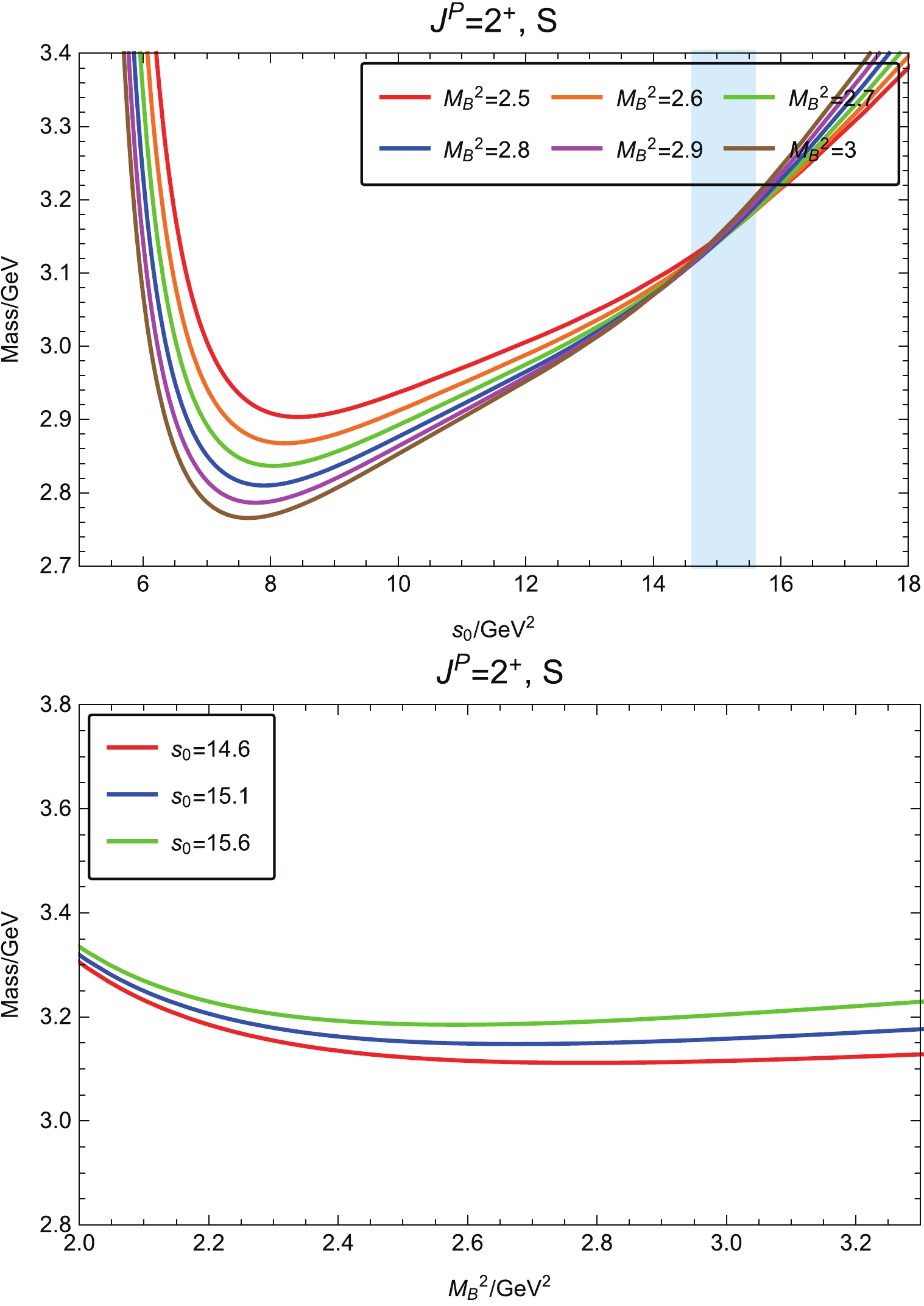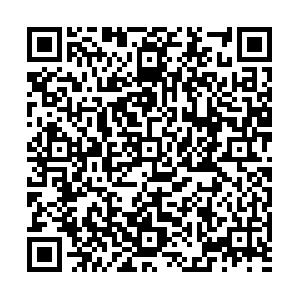# Exotic ΩΩ dibaryon states in a molecular picture• We investigate the exotic $\Omega\Omega$dibaryon states with $J^P=0^+$and $2^+$in a molecular picture. We construct a tensor $\Omega$$\Omega$molecular interpolating current and calculate the two-point correlation function within the method of QCD sum rules. Our calculations indicate that the masses of the scalar and tensor dibaryon states are $m_{\Omega\Omega, \, 0^+} =$$(3.33\pm 0.51) \,{\rm{GeV}}$and $m_{\Omega\Omega,\, 2^+}=(3.15\pm0.33)\, {\rm{GeV}}$, respectively, which are below the $2m_\Omega$threshold. Within error, these results do not negate the existence of loosely bound molecular $\Omega\Omega$dibaryon states. These exotic strangeness $S=-6$and doubly-charged $\Omega\Omega$dibaryons, if they exist, may be identified in heavy-ion collision processes in the future.
••Get Citation
Xiao-Hui Chen, Qi-Nan Wang, Wei Chen and Hua-Xing Chen. Exotic ΩΩ dibaryon states in a molecular picture[J]. Chinese Physics C. doi: 10.1088/1674-1137/abdfbe
Xiao-Hui Chen, Qi-Nan Wang, Wei Chen and Hua-Xing Chen. Exotic ΩΩ dibaryon states in a molecular picture[J]. Chinese Physics C.Milestone
Received: 2020-11-22
Article Metric

Article Views(280)
PDF Downloads(29)
Cited by(0)
Policy on re-use
To reuse of Open Access content published by CPC, for content published under the terms of the Creative Commons Attribution 3.0 license (“CC CY”), the users don’t need to request permission to copy, distribute and display the final published version of the article and to create derivative works, subject to appropriate attribution.
###### 通讯作者: 陈斌, bchen63@163.com
• 1.

沈阳化工大学材料科学与工程学院 沈阳 110142

Title:
Email:

## Exotic ΩΩ dibaryon states in a molecular picture

###### Corresponding author: Hua-Xing Chen, hxchen@seu.edu.cn
• 1. School of Physics, Sun Yat-Sen University, Guangzhou 510275, China
• 2. School of Physics, Southeast University, Nanjing 210094, China

Abstract: We investigate the exotic $\Omega\Omega$dibaryon states with $J^P=0^+$and $2^+$in a molecular picture. We construct a tensor $\Omega$$\Omega$molecular interpolating current and calculate the two-point correlation function within the method of QCD sum rules. Our calculations indicate that the masses of the scalar and tensor dibaryon states are $m_{\Omega\Omega, \, 0^+} =$$(3.33\pm 0.51) \,{\rm{GeV}}$and $m_{\Omega\Omega,\, 2^+}=(3.15\pm0.33)\, {\rm{GeV}}$, respectively, which are below the $2m_\Omega$threshold. Within error, these results do not negate the existence of loosely bound molecular $\Omega\Omega$dibaryon states. These exotic strangeness $S=-6$and doubly-charged $\Omega\Omega$dibaryons, if they exist, may be identified in heavy-ion collision processes in the future.

### HTMLII.   QCD SUM RULES FOR DIBARYON SYSTEMSIII.   PREDICTION FOR THE SCALAR $\Omega\Omega$ DIBARYON WITH ${J^P = 0^+}$IV.   PREDICTION FOR THE TENSOR ${\Omega\Omega}$ DIBARYON WITH ${J^P = 2^+}$APPENDIX
Reference (49)
PDF查看关注分享

Top

### 目录/DownLoad:  Full-Size Img  PowerPoint